# Equations with fractions

## Summary and examples

Roughly speaking an equation with fractions is an equation with the independent variable (for example) appearing in the denominator or in both the numerator and denominator.

In a number of examples we clarify how to solve equations with fractions.

##### Example 1

Solve: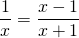The equation is only defined for denominators unequal to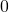: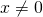andEssential in this first method is that fractions can only be added (or subtracted) if they have equal denominators. That is why we make both denominators equal: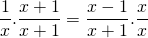resulting in the equation: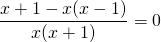or: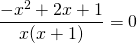or: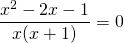A fraction is equal toif the numerator is equal to, thus:This equation is solved by applying the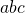-formula: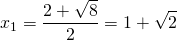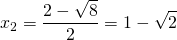A second method (cross-multiplication) is straightforward. Cross-multiplication means that the product ofand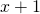is made equal to the productand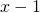, thus: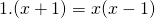resulting in the same equation.

Usually cross-multiplication is preferred because it is a faster method. However, this method fails if we have inequalities with fractions. We come back to this in detail in Inequalities with fractions.

##### Example 2

Solve: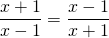The equation is only defined for denominators unequal to: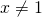andCross-multiplication yields: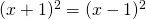or: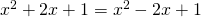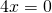so: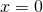##### Example 3

Solve: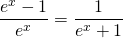Both denominators are not equal tofor all values of, and thus the equation is defined for alle values of.

Cross-multiplication yields: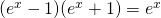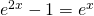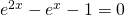This is a quadratic equation in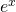and the solution is (using the-formula):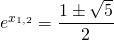Just one of the two values is positive and thus valid since a power function cannot be negative. So: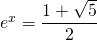and thus, after having applied the natural logarithm: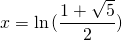##### Example 4

Solve: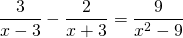The equation is only valid if the denominators are unequal to: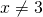en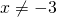In order to solve this equation we have to make the denominators in the left-hand side equal: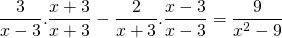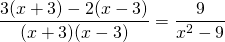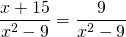and thus: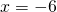0